# Graph theory

(diff) ← Older revision | Latest revision (diff) | Newer revision → (diff)

A branch of discrete mathematics, distinguished by its geometric approach to the study of various objects. The principal object of the theory is a graph and its generalizations. The first problems in the theory of graphs were solutions of mathematical puzzles (the problem of the bridges of Königsberg, the disposition of queens on a chessboard, transportation problems, the travelling-salesman problem, etc.). One of the first results in graph theory was a criterion on the possibility of traversing all edges of a graph without passing through any edge more than once; it was obtained by L. Euler in 1736 in solving the problem of the bridges of Königsberg. The four-colour problem, formulated in the mid-19th century, though a mere amusement puzzle at first sight, led to studies of graphs of both theoretical and applied interest. Certain studies in the mid-19th century contain results of importance to graph theory, obtained by solving practical problems. Thus, G. Kirchhoff's complete set of equations  for currents and voltages in electric circuits really amounts to representing the circuit by a graph with skeleton trees, with the aid of which linearly independent circuit systems are obtained. A. Cayley , starting from the problem of calculating the number of isomers of saturated hydrocarbons, arrived at the problems of listing and describing trees with certain properties, and solved some of them. In the 20th century, problems involving graphs began to arise not only in physics, chemistry, electrical engineering, biology, economics, sociology, etc., but also in mathematics itself — in topology, algebra, probability theory, and number theory. At the beginning of the 20th century, graphs were used to represent certain mathematical objects and to formally state various discrete problems; besides the term "graph" , other terms such as map, complex, diagram, network, labyrinth, were also employed. Following the appearance of the monograph of D. König  in 1936, the term "graph" has been used more frequently than other terms. This study reviewed the facts known at that time. The first results, concerning connectivity properties, planarity, and graph symmetry, which paved the way for a number of novel directions of study in graph theory, appeared in the 1920s and 1930s. The scope of research in graph theory was considerably extended in the late 1940s and early 1950s, mainly as a result of the development of cybernetics and calculation techniques. Interest in graph theory increased, and the range of problems dealt with by the theory was considerably extended. The use of electronic computers made it possible to solve practical problems involving extensive calculations, which could not be solved previously. Methods were developed for solving a number of extremal problems in graph theory; one such problem is the construction of the maximum flow across a network (cf. Flow in a network). It was shown, for individual classes of graphs (trees, planar graphs, etc.), which had been studied before, that the solution of certain problems was simpler for such graphs than for arbitrary graphs (finding conditions for the existence of graphs with certain properties, establishment of graph isomorphism, etc.).

Some problems in graph theory are more combinatorial in nature, while others are essentially geometric. The former include, for example, the calculation and listing of graphs with prescribed properties and the construction of graphs with prescribed properties. Many types of problems, such as on graph circuits (cf. Graph circuit) and graph imbedding, are of geometric (topological) nature. Certain problems concern the modes of classification of graphs, e.g. classification by the properties of partitions of them. Results concerning the existence of graphs with certain properties may be exemplified by the criterion of realizability of numbers by the degrees of the vertices of a given graph: A collection of integers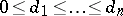, the sum of which is even, can be realized by degrees of the vertices of a graph without loops and multiple edges if and only if the condition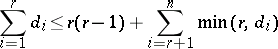is fulfilled for any().

Problems of the enumeration of graphs with prescribed properties can be exemplified by problems of finding the number of non-isomorphic graphs with the same number of vertices and/or edges. The number of non-isomorphic trees withvertices is given by the asymptotic formulawhere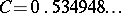,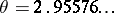. The number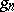of non-isomorphic graphs without loops or multiple edges and withvertices is given by the formula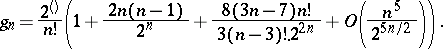Graph theory deals with specific types of problems, as well as with problems of a general nature. One type of such specific problems is the connectivity of graphs, and the study of the structure of a graph based on its connectivity (cf. Graph, connectivity of a). In the analysis of the reliability of electronic circuits or communications networks there arises the problem of finding the number of non-intersecting chains connecting the various vertices of a graph. Here, a number of results have been obtained. Thus, the smallest number of vertices separating two non-adjacent vertices of a graph is equal to the greatest number of non-intersecting (at the vertices) simple chains which connect this pair of vertices. Criteria have been found and effective algorithms have been developed for the establishment of the degree of connectivity of a graph (the smallest number of vertices or edges the removal of which destroys the connectedness of the graph).

Another direction of study in graph theory is the investigation of walks (edge progressions) containing all the vertices or all the edges of a graph (cf. Graph circuit). A simple criterion for the existence of a walk containing all the edges of a graph is available: In a connected graph a cycle containing all the edges and passing through each edge once and only once exists if and only if the degrees of all except two vertices of the graph are even. If the set of vertices of a graph is traversed, only a number of sufficient conditions for the existence of a cycle passing through each vertex once is available.

A characteristic type of problems dealt with by graph theory concerns graph colouring. They involve the partition of the set of vertices (edges) having certain properties, e.g. adjacent vertices (edges) are to belong to different sets (vertices or edges of the same set are drawn with the same colour; cf. Graph colouring). It has been proved that the least number of colours required for colouring the edges of any graph without loops of maximum degreeis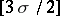, while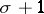colours are sufficient for the colouring of the vertices of any graph without loops or multiple edges.

Other types of problems also exist (cf. Covering and packing; Graph imbedding; Graph, numerical characteristics of a); some of them were suggested by other branches of mathematics. Thus, topology initiated the study of imbedding graphs in various surfaces. For example, the following condition is necessary and sufficient for the imbedding of a graph in a plane (the Pontryagin–Kuratowski criterion): A graph is planar (cf. Graph, planar) if and only if it contains no subgraphs obtained by partitioning of the edges of the complete-vertex graph, or the complete bipartite graph, with three vertices in each part. Algebra initiated the study of graph automorphisms (cf. Graph automorphism). It has been shown, in particular, that any finite group is isomorphic to the automorphism group of some graph. Probability theory stimulated the study of random graphs (cf. Graph, random). Many properties have been studied for "almost-all" graphs; it has been shown, for example, that almost-all graphs withvertices are connected, that their diameter is 2, and that they possess a Hamilton cycle (a cycle passing through each vertex of the graph once).

Graph theory comprises specific methods for solving extremal problems. One such method is based on the max-flow-min-cut theorem, which states that the maximum flow which can pass through a network from a vertexto a vertexequals the minimum transmission capacity of the cuts separating the verticesand(cf. Flow in a network). Various effective algorithms for finding the maximum flow have been developed.

Algorithmic problems are of major importance in graph theory. For finite graphs, i.e. for graphs with a finite number of vertices and edges, an algorithm for a solution of problems, including extremal problems, usually exists. Many problems involving finite graphs can be solved by complete inspection of all admissible variants. However, such a method solves the problem only for graphs with a small number of vertices and edges. It is therefore highly important in graph theory to construct effective algorithms by means of which an exact or an approximate solution can be found. Such algorithms have in fact been constructed for certain problems, such as the establishment of planarity of graphs, the determination of isomorphism of trees, or the finding of the maximum flow.

The results and methods of graph theory are used for solving transportation problems, to find optimal solutions for the assignment problem, to identify bottlenecks in the planning and control of project development, in establishing the optimum routes for the supply of goods, as well as in modelling complex technological processes, in the construction of various discrete mechanisms, in programming, etc.

How to Cite This Entry:
Graph theory. Encyclopedia of Mathematics. URL: http://encyclopediaofmath.org/index.php?title=Graph_theory&oldid=15471
This article was adapted from an original article by V.B. AlekseevV.P. KozyrevA.A. Sapozhenko (originator), which appeared in Encyclopedia of Mathematics - ISBN 1402006098. See original article